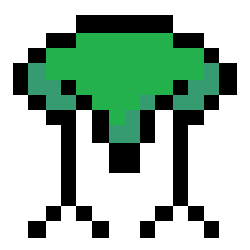# 🍉 In JS, is an empty Array return false or true?

May 15, 2018 • ☕️ 2 min read

## 😃 General

When I was coding, there was a situation that if an array has values, it will return true. So I wrote like this `bool = !!array`. But, unexpectedly when `array = []`, `bool` is `false`, not `true`.

## 🤔 Why?

• First, I thought if empty `string` or empty `Array` is conversed to `Boolean`, it must return `false`.
• But it is not.
``````// Conversion
> Boolean("")
false
> Boolean([])
true
// Implied Conversion
> !!""
false
> !![]
true``````
• In Conversion, the result is like this, so I thought `''` is `false`, `[]` is `true`.
``````// Loose Equality
> "" == false
true
> [] == false
true``````
• But here in Loose Equality, the result is `''` is `false`(of course), `[]` is `false` too.
• In Loose Equality, `[]` is `Object`, and `false` is `Boolean`. So in the table, it is applied that `ToPrimitive([]) == ToNumber(false)` and recursively `ToNumber("") === 0`. Eventually, it returns true.
• I certainly realized when I saw this table. plz memorize it.

#### toBoolean Table

Argument Type Result
`undefined` `false`
`null` `false`
`boolean` same as input
`number` `+0`, `-0`, `NaN` -> `false`, otherwise -> `true`
`string` empty string -> `false`, otherwise -> `true`
`object` `true`

### 🤖 Conclusion

• Because `Array` is type of `object`, the fact that an empty `Array` is conversed to `true` is correct.
• But in Loose Equality, `[] == false` is right.
• So, above `bool = !!array` code can be changed `bool = array == false`.
• Or, you can make function that checks `array === undefined` or `array.length === 0`. 🥝

### blog.holy.kiwiPersonal blog by Jon Jee.
I hope to explain with words and code.